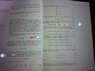# Separable PDE for electric field in a cavity

• gboff21
In summary, the conversation discusses a photo from a book on Laser Physics and three questions related to it. The questions address the notation of "c.c." in the book, why the vector function U is separated into Ux, Uy, and Uz, and the presence of the term \frac{\pi}{L} in the solution of a PDE. The conversation also includes a clarification on the meaning of "c.c." and the use of L in the equation. The origin of the cos function in the solution is also mentioned, although it remains unclear.

## Homework Statement

Here is a photo of a page in Laser Physics by Hooker:
https://www.evernote.com/shard/s245/sh/2172a4e7-63c7-41a0-a0e7-b1d68ac739fc/7ba12c241f76a317a6dc3f2d6220027a/res/642710b5-9610-4b5b-aef4-c7958297e34d/Snapshot_1.jpg?resizeSmall&width=832

I have 3 questions (I'm a bit rusty after a long summer):
1. Is the "c.c." bascially $\textbf{U}(r) e^{i \omega t}$ or is the U actually U*? (Just checking)

2. Why can't we say $\textbf{U}(r) = X(x)Y(y)Z(z)$ instead of separating it into Ux Uy and Uz?

3. Finally: Having gone through the PDE and got an answer with boundary solutions $\textbf{U}(r)_{x} = 0$ at edges, I don't understand where the $\frac{\pi}{L}$ comes from. The π I know is just put into make the mode numbers simpler later, but how does the L get there?
And how is the x component a cos function? I get a sin!

Thanks for the help! I'm just a bit rusty!

Oh, ok here you go (attached)

#### Attachments

•Snapshot_1.jpg
48.3 KB · Views: 407
the +c.c. simply means "plus the conjugate complex expression", i.e.,
$$a+ \text{c.c.}=a+a^*.$$

•1 person

1: Yes
2: Note: U (a 3D vector) is a function of r (which contains x, y, and z.)
3: L is the cavity size (some times you will see 2*Pi/L) depending on boundary conditions and such.

Hope this helps!

As for the cos, I am unsure about this as well. Perhaps someone else can shed some light on this matter.

Last edited:
•1 person